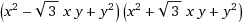# Proving a Trigonometric Identity

## Homework Statement

Prove that:

$\cos^6{(x)} + \sin^6{(x)} = \frac{5}{8} + \frac{3}{8} \cos{(4x)}$

## Homework Equations

I am not sure. I used factoring a sum of cubes.

## The Attempt at a Solution

I tried $\cos^6{(x)} + \sin^6{(x)} = \cos^4{(x)} - \cos^2{(x)} \sin^2{(x)} + \sin^4{(x)}$. But I can't get anywhere beyond this; I must be missing something obvious.

## Answers and Replies

HallsofIvy
Science Advisor
Homework Helper
Sounds good to me! Now, you might try factoring "$cos^2(x)$ out of the first two terms: $cos^2(x)(cos^2(x)- sin^2(x))+ sin^4(x)= cos^2(x)cos(2x)- sin^4(x)$ see where you can go from that.

Simon Bridge
Science Advisor
Homework Helper
Id normally just throw the euler formula at these things ... unless I had an already proved identity I could use.

SammyS
Staff Emeritus
Science Advisor
Homework Helper
Gold Member
Although, x2 - xy + y2 cannot be factored (over the reals), x4 - x2 y2 + y4 can be factored .#### Attachments

SteamKing
Staff Emeritus
Science Advisor
Homework Helper
Rather than attacking the LHS of the identity, I would prefer to look at the expression cos (4x) instead. The multiple angle formulas for cosine I think would be more helpful here than trying to factor polynomials.

haruspex
Science Advisor
Homework Helper
Gold Member
2020 Award
The 4x on the right, and the 8s in the denominators, are strong clues. Do you know how to expand cos(2x) in terms of cos(x) and sin(x)? Just apply that (in reverse) a couple of times.

Edit... SteamKing's (equivalent) post wasn't there when I hit reply, even though it seems to have been made hours earlier. Strange.